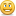# Progress indicator using variables question

I am creating 6 questions in which there will be an arrow pointing to different levels depending on whether they get the questions right or wrong.  I've been using true/false variables to make this work.  However, for each additional question, the number of true/false combinations are getting exponentially higher.  Is there a simpler method using non true/false variables?

I have attached an image of the progress indicator where the arrow turns to visible depending on whether they got the question right or wrong.

Thanks as always!

Jay

###### 3 RepliesIf I understand correctly, you have 6 questions of which answer is assigned with true/false value. All of the true/false answers combine together determines the position of the arrow on the expertise level bar. (For instance, in the situation where all questions are answered correctly, the arrow points to Guru.)

If so, we can do a little math here. There are 6 questions, then there will be 2*2*2*2*2*2 = 64 combinations of answers. And yeah, it can be overwhelmingMy suggestion is use a numeric variable and let the value of the variable to determine the position of the arrow on the bar.

Here is how: create a numeric variable "pointvalue", and assign the default value as 0. So every time a questions is answered correctly, add 1 to "valuepoint", and when answer is wrong, add 0 to "valuepoint". The value of the "valuepoint" determines the level of expertise on the bar, like 6 means the top of the bar, and 0 means the bottom.

Hope it helps

Cheng